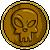# Non Linear Sim Eqs

3 replies to this topic

### #1jacci

jacci

Newbie

•• Members
•• 3 posts
• Gender:Female

• Calculators:
fx82au
fx115es

Posted 02 May 2008 - 02:51 PM

Hi, I know this is actually not that hard to do by hand, or to manipulate the equation to make it into simple linear equations which i can then solve. I am new to the classpad though and am trying to get my head around the basic functions and how to make them work.

I am trying to solve

52x-y=625
2x-y=32

I have tried solving using the 2d template and the solve( function and i am getting the solution returned:
{52x-y=625,2x-y=32}

When I transform into two linear equations: 2x - y = 4 and x - y = 5, i get the correct result.

this is more an exercise for me in learning the classpad than an actual math problem, so if anybody knows how i can use the calc to solve this, (or tell me it cant be done) then i would be very grateful.

### #2Colombia

Colombia

•• Members
•• 94 posts
• Gender:Male
• Location:Venezuela

• Calculators:
algebra FX 2.0 plus

Posted 03 May 2008 - 03:06 AM

The classpad is just a maquine you need to think how to make you calculus easier.

where C is a constant.

### #3jacci

jacci

Newbie

•• Members
•• 3 posts
• Gender:Female

• Calculators:
fx82au
fx115es

Posted 06 May 2008 - 12:14 AM

thanks, I thought as much, just checking the limits of what it can do. Still in the early exploring stages of what the classpad is capable of

### #4Behnoud

Behnoud

Casio Technician

•• Members
•• 338 posts
• Gender:Male
• Location:Iran

• Calculators:
CP 300+ OS2.2/
FX 3600 pv/
FX 115 s/
FX 602P

Posted 11 May 2008 - 04:52 PM

As I experienced, it take less to sovle y*Ln(x)=C than x^y=C!
And using 2D make expression clear but it's too time consuming to solve the complicated
2D equations.

#### 0 user(s) are reading this topic

0 members, 0 guests, 0 anonymous users